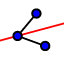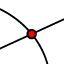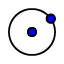# Angle Bisectors and Incenters

## Introduction

An incenter of a triangle is found at the intersection of the angle bisectors of a triangle. These exercises explore constructing angle bisectors, incenters, and their properties.

## Constructing Angle Bisectors

*A note before we begin. In these exercises, we will use some of the shortcut methods that Geogebra has to offer in order to do our constructions.* In the exercise below construct the angle bisector of angle ABC. To do this, we will use the Angle Bisector tool. This tool is found under the Perpendicular Line tool## Property of Angle Bisectors

Ray BP is the angle bisector of angle ABC below. Points K and L lie on perpendiculars to the sides of the angles that go through point P. Move point P about on the ray and notice how the lengths of PK and PL change. Then answer the question that follows.

What can you say about the distance from a point that lies on a perpendicular bisector to each of the sides of the angle?

## Constructing the Incenter

In the exercise below, find the incenter by constructing the angle bisector for two of the angles of the triangle. Then create a point at the intersection of the angle bisectors using the Intersect toolwhich is found under the Point menu. Next construct the inscribed circle. The incenter is the center of an inscribed circle in a triangle. First, you need to construct the perpendicular line to one side of the triangle that goes through your incenter. To do this, select the Perpendicular Line tool, then click on your incenter and then side AB of the triangle. Next, create a point at the intersection of this perpendicular and side AB using the Intersect tool. Now, select the Circle with Center Through a Point tooland click on the incenter, and then the intersection you just created on side AB.

What are the approximate coordinate of the incenter (don't worry about being completely exact, just round to the nearest tenth or so. Also recall that the incenter MUST be inside the circle)

What is the incenter equidistant to?